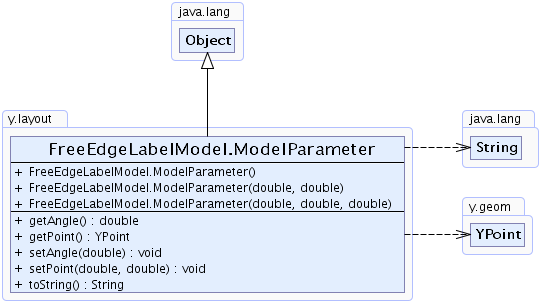Search this API

## y.layout Class FreeEdgeLabelModel.ModelParameter

```java.lang.Objecty.layout.FreeEdgeLabelModel.ModelParameter
```
Enclosing class:
FreeEdgeLabelModel

`public static class FreeEdgeLabelModel.ModelParameterextends java.lang.Object`

This model parameter encodes a label position within `FreeEdgeLabelModel`. It represents every possible location in relation to the source point of the edge. The radius describes the distance of the label center from the source point. There is an angle `theta` which defines the location on the circle with the given radius. It is the angle between the first segment of the underlying edge and the imaginary line to the center of the label. Finally, there is a second angle that describes the rotation of the label box.

`setPoint(double, double)`, `setAngle(double)`Constructor Summary
`FreeEdgeLabelModel.ModelParameter()`
Creates a new instance of `FreeEdgeLabelModel.ModelParameter` with radius `50` and angle `0`.
```FreeEdgeLabelModel.ModelParameter(double radius, double theta)```
Creates a new instance of `FreeEdgeLabelModel.ModelParameter` with the given radius and angle.
```FreeEdgeLabelModel.ModelParameter(double radius, double theta, double angle)```
Creates a new instance of `FreeEdgeLabelModel.ModelParameter` with the given radius, angle and rotation angle.

Method Summary
` double` `getAngle()`
Returns the rotation angle of the label that is placed using this model parameter.
` YPoint` `getPoint()`
Returns a point that represents the location of the label's center in relation to the source point of the edge.
` void` `setAngle(double angle)`
Specifies the rotation angle of the label that is placed using this model parameter.
` void` ```setPoint(double radius, double theta)```
Specifies a point that represents the location of the label's center in relation to the source point of the edge.
` java.lang.String` `toString()`
Returns a `String` representation of this model parameter.

Methods inherited from class java.lang.Object
`clone, equals, finalize, getClass, hashCode, notify, notifyAll, wait, wait, wait`

Constructor Detail

### FreeEdgeLabelModel.ModelParameter

`public FreeEdgeLabelModel.ModelParameter()`
Creates a new instance of `FreeEdgeLabelModel.ModelParameter` with radius `50` and angle `0`.

### FreeEdgeLabelModel.ModelParameter

```public FreeEdgeLabelModel.ModelParameter(double radius,
double theta)```
Creates a new instance of `FreeEdgeLabelModel.ModelParameter` with the given radius and angle.

Parameters:
`radius` - the distance of the label from the source node of the underlying edge
`theta` - the angle in radians between the first segment of the underlying edge and the imaginary line to the center of the label

### FreeEdgeLabelModel.ModelParameter

```public FreeEdgeLabelModel.ModelParameter(double radius,
double theta,
double angle)```
Creates a new instance of `FreeEdgeLabelModel.ModelParameter` with the given radius, angle and rotation angle.

Parameters:
`radius` - the distance of the label from the source node of the underlying edge
`theta` - the angle in radians between the first segment of the underlying edge and the imaginary line to the center of the label
`angle` - the rotation angle of the label
Method Detail

### getAngle

`public double getAngle()`
Returns the rotation angle of the label that is placed using this model parameter.

Returns:
the rotation angle of the label
`setAngle(double)`

### setAngle

`public void setAngle(double angle)`
Specifies the rotation angle of the label that is placed using this model parameter.

Default Value:
The default value is 0. The label is placed paraxial.
Parameters:
`angle` - the rotation angle of the label

### getPoint

`public YPoint getPoint()`
Returns a point that represents the location of the label's center in relation to the source point of the edge. The x-coordinate corresponds to the radius which describes the distance of the label to the source point. The y-coordinate corresponds to the angle between the first segment of the underlying edge and the imaginary line to the center of the label.

Returns:
the location of the label in relation to the edge's source point

### setPoint

```public void setPoint(double radius,
double theta)```
Specifies a point that represents the location of the label's center in relation to the source point of the edge. The x-coordinate corresponds to the radius which describes the distance of the label to the source point. The y-coordinate corresponds to the angle between the first segment of the underlying edge and the imaginary line to the center of the label.

Parameters:
`radius` - the distance to the source point
`theta` - the location on the radius

### toString

`public java.lang.String toString()`
Returns a `String` representation of this model parameter. The `String` contains the radius around the source node on which the label is placed and the angle that describes the location on said radius.

Overrides:
`toString` in class `java.lang.Object`
Returns:
the `String` representation